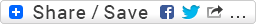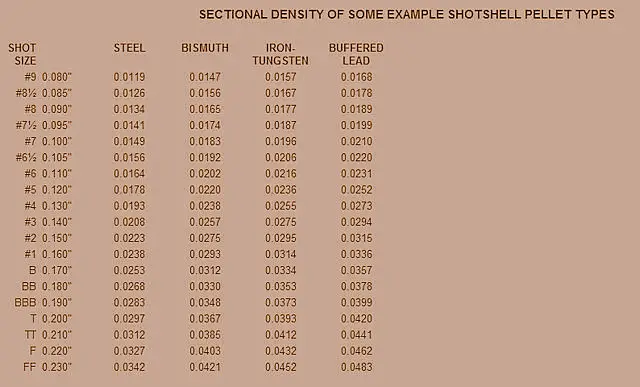Shotshell Pellet Ballistic Coefficients and Sectional Density by Ed LowryA shotshell pellet's ballistic coefficient is a comparative measure of its ability to overcome the force of air resistance. It is numerically equal to the pellet's sectional density divided by a so called form factor, which is an index of pellet shape. The pellet's sectional density is equal to its weight (in lbs.) divided by the the square of its diameter (in inches). The form factor for a truly spherical pellet is equal to one (1.0). Hence, for an undeformed, spherical pellet, the ballistic coefficient is simply equal to its sectional density. If two dissimilar pellets have the same ballistic coefficient and are launched with the same muzzle velocity, their velocities (and flight times) at any downrange point will be the same. Thus, as seen in the table below, a #5 steel pellet and a Buffered Lead #8-1/2 pellet will have identical downrange velocities (and flight times) if they are each of equal roundness in flight and if each is launched with the same muzzle velocity.Copyright 2013 by Randy Wakeman. All Rights Reserved.Custom Search